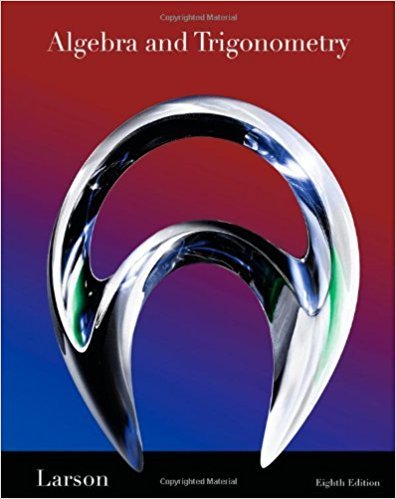×
×

# Solutions for Chapter 5.3: Properties of Logarithms## Full solutions for Algebra and Trigonometry | 8th Edition

ISBN: 9781439048474Solutions for Chapter 5.3: Properties of Logarithms

Solutions for Chapter 5.3
4 5 0 256 Reviews
24
1
##### ISBN: 9781439048474

Since 115 problems in chapter 5.3: Properties of Logarithms have been answered, more than 47332 students have viewed full step-by-step solutions from this chapter. This textbook survival guide was created for the textbook: Algebra and Trigonometry, edition: 8. This expansive textbook survival guide covers the following chapters and their solutions. Chapter 5.3: Properties of Logarithms includes 115 full step-by-step solutions. Algebra and Trigonometry was written by and is associated to the ISBN: 9781439048474.

Key Math Terms and definitions covered in this textbook
• Factorization

A = L U. If elimination takes A to U without row exchanges, then the lower triangular L with multipliers eij (and eii = 1) brings U back to A.

• Four Fundamental Subspaces C (A), N (A), C (AT), N (AT).

Use AT for complex A.

• Fourier matrix F.

Entries Fjk = e21Cijk/n give orthogonal columns FT F = nI. Then y = Fe is the (inverse) Discrete Fourier Transform Y j = L cke21Cijk/n.

• Free variable Xi.

Column i has no pivot in elimination. We can give the n - r free variables any values, then Ax = b determines the r pivot variables (if solvable!).

• Hessenberg matrix H.

Triangular matrix with one extra nonzero adjacent diagonal.

• Hypercube matrix pl.

Row n + 1 counts corners, edges, faces, ... of a cube in Rn.

• Independent vectors VI, .. " vk.

No combination cl VI + ... + qVk = zero vector unless all ci = O. If the v's are the columns of A, the only solution to Ax = 0 is x = o.

• Kirchhoff's Laws.

Current Law: net current (in minus out) is zero at each node. Voltage Law: Potential differences (voltage drops) add to zero around any closed loop.

• Least squares solution X.

The vector x that minimizes the error lie 112 solves AT Ax = ATb. Then e = b - Ax is orthogonal to all columns of A.

• Left inverse A+.

If A has full column rank n, then A+ = (AT A)-I AT has A+ A = In.

• Multiplication Ax

= Xl (column 1) + ... + xn(column n) = combination of columns.

• Right inverse A+.

If A has full row rank m, then A+ = AT(AAT)-l has AA+ = 1m.

• Row picture of Ax = b.

Each equation gives a plane in Rn; the planes intersect at x.

• Saddle point of I(x}, ... ,xn ).

A point where the first derivatives of I are zero and the second derivative matrix (a2 II aXi ax j = Hessian matrix) is indefinite.

• Schur complement S, D - C A -} B.

Appears in block elimination on [~ g ].

• Simplex method for linear programming.

The minimum cost vector x * is found by moving from comer to lower cost comer along the edges of the feasible set (where the constraints Ax = b and x > 0 are satisfied). Minimum cost at a comer!

• Skew-symmetric matrix K.

The transpose is -K, since Kij = -Kji. Eigenvalues are pure imaginary, eigenvectors are orthogonal, eKt is an orthogonal matrix.

• Solvable system Ax = b.

The right side b is in the column space of A.

• Standard basis for Rn.

Columns of n by n identity matrix (written i ,j ,k in R3).

• Unitary matrix UH = U T = U-I.

Orthonormal columns (complex analog of Q).

×